Vivian 2018-06-06 来源 ： 阅读 166 评论 0

```a = ['scolia', 123, [], ]
b = a[:]
b.append(666)
print a
print b``````a = ['scolia', 123, [], ]
b = a[:]
print id(a), id(a), id(a), id(a)
print id(b), id(b), id(b), id(b)``````a = ['scolia', 123, [], ]
b = a[:]
b = 666
print a
print b``````a = ['scolia', 123, [], ]
b = a[:]
b = 666
print id(a), id(a), id(a), id(a)
print id(b), id(b), id(b), id(b)```1.切片操作

2.使用list()工厂函数新建对象。（ b = list(a) ）```from copy <d>import</d> deepcopy
a = ['scolia', 123, [], ]
b = deepcopy(a)
b = 666
print id(a), id(a), id(a), id(a)
print id(b), id(b), id(b), id(b)```1.正如代码示例用一样，只能通过内置的copy模块的deepcopy()方法创建。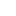喜欢 | 1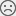不喜欢 | 0

JAVA工程师交流群 +加入

WEB/H5前端交流群 +加入•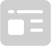资料索取
•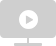答疑解惑
•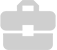技术交流
•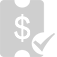职业测评
•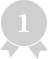面试技巧
•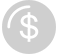高薪秘籍
Python30天热搜词 更多>>
X